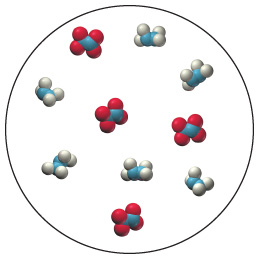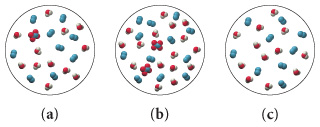# Problem: Consider the following reaction: 2N2H4 (g) + N2O4 (g) → 3N2(g) + 4H2O(g) Consider the following representation of an initial mixture of N2H4 and N2O4 : Which of the following best represents the reaction mixture after the reactants have reacted as completely as possible?

🤓 Based on our data, we think this question is relevant for Professor Bronikowski's class at UT.

###### FREE Expert Solution

Balanced reaciton:

2 N2H4 (g) + N2O4 (g) → 3N2(g) + 4H2O(g)

To have a proper visualization, we must assign the colors to specific atoms so that we know what we are looking at.

Blue = N (Since it is in the middle of the molecules)
White = H (slightly smaller)
Red = O###### Problem Details

Consider the following reaction: 2N2H4 (g) + N2O4 (g) → 3N2(g) + 4H2O(g) Consider the following representation of an initial mixture of N2H4 and N2O4 :Which of the following best represents the reaction mixture after the reactants have reacted as completely as possible?